﻿ 热传导方程初边值问题的三次样条解 Cubic Spline Solution to Initial Boundary Value Problems of Heat Transfer Equations

Vol. 08  No. 08 ( 2019 ), Article ID: 31696 , 9 pages
10.12677/AAM.2019.88161

Cubic Spline Solution to Initial Boundary Value Problems of Heat Transfer Equations

Dan Liu, Tianjun Wang

College of Mathematics and Statistics, Henan University of Science and Technology, Luoyang Henan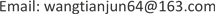Received: Jul. 20th, 2019; accepted: Aug. 7th, 2019; published: Aug. 14th, 2019ABSTRACT

In order to investigate the numerical solution approximated to the exact solution of the initial boundary value problems of the heat transfer equations, the cubic spline solution is given here. Using the parametric cubic spline function based on Legendre-Gauss-Lobatto node for the numerical solution of Dirichlet boundary value problems of the heat transfer equations, the spatial direction is discretized by cubic spline function with parameters, and the parameters are appropriately chosen to improve accuracy of numerical errors and use the Crank-Nicolson format to construct the algorithm scheme in the time direction. Finally, the algorithm and numerical examples are given to demonstrate the high efficiency and accuracy of the proposed algorithm.

Keywords:Heat Transfer Equation, Initial Boundary Value Problems, Legendre-Gauss-Lobatto Nodes, Parametric Cubic Spline Function1. 引言

2. 算法格式

2.1. Legendre-Gauss-Lobatto节点

n次Legendre多项式的定义如下：

${L}_{n}\left(t\right)=\frac{{\left(-1\right)}^{n}}{{2}^{n}n!}\frac{{\text{d}}^{n}\left({\left(1-{t}^{2}\right)}^{n}\right)}{\text{d}{t}^{n}},n=0,1,\cdots ,t\in \left[-1,1\right].$

2.2. 含参数的三次样条函数

${S}^{″}\left(x\right)+\xi S\left(x\right)=\left({S}^{″}\left({x}_{i}\right)+\xi S\left({x}_{i}\right)\right)\frac{{x}_{i+1}-x}{{h}_{i+1}}+\left({S}^{″}\left({x}_{i+1}\right)+\xi S\left({x}_{i+1}\right)\right)\frac{x-{x}_{i}}{{h}_{i+1}},i=0,1,\cdots ,n-1.$ (1)

$S\left(x\right)={C}_{1}\mathrm{cos}\sqrt{\xi }x+{C}_{2}\mathrm{sin}\sqrt{\xi }x.$

${S}^{*}\left(x\right)=\left({S}^{″}\left({x}_{i}\right)+\xi S\left({x}_{i}\right)\right)\frac{{x}_{i+1}-x}{\xi {h}_{i+1}}+\left({S}^{″}\left({x}_{i+1}\right)+\xi S\left({x}_{i+1}\right)\right)\frac{x-{x}_{i}}{\xi {h}_{i+1}}.$

${\omega }_{i+1}={h}_{i+1}{\xi }^{1/2}$ ，再由插值条件： $S\left({x}_{i}\right)={u}_{i},S\left({x}_{i+1}\right)={u}_{i+1}$ ，则可以得到公式(1)的通解，如下：

$\begin{array}{c}S\left(x\right)=-\frac{{h}_{i+1}^{2}}{{\omega }_{i+1}^{2}\mathrm{sin}{\omega }_{i+1}}\left[{S}^{″}\left({x}_{i+1}\right)\mathrm{sin}\left(\frac{{\omega }_{i+1}\left(x-{x}_{i}\right)}{{h}_{i+1}}\right)+{S}^{″}\left({x}_{i}\right)\mathrm{sin}\left(\frac{{\omega }_{i+1}\left({x}_{i+1}-x\right)}{{h}_{i+1}}\right)\right]\\ \text{\hspace{0.17em}}\text{\hspace{0.17em}}+\frac{{h}_{i+1}^{2}}{{\omega }_{i+1}^{2}}\left[\frac{x-{x}_{i}}{{h}_{i+1}}\left({S}^{″}\left({x}_{i+1}\right)+\frac{{\omega }_{i+1}^{2}}{{h}_{i+1}^{2}}S\left({x}_{i+1}\right)\right)+\frac{{x}_{i+1}-x}{{h}_{i+1}}\left({S}^{″}\left({x}_{i}\right)+\frac{{\omega }_{i+1}^{2}}{{h}_{i+1}^{2}}S\left({x}_{i}\right)\right)\right].\end{array}$ (2)

${S}^{\prime }\left({x}_{i}+0\right)=-\frac{{S}^{″}\left({x}_{i+1}\right)-{S}^{″}\left({x}_{i}\right)\mathrm{cos}{\omega }_{i+1}}{{\xi }^{1/2}\mathrm{sin}{\omega }_{i+1}}+\frac{{S}^{″}\left({x}_{i+1}\right)-{S}^{″}\left({x}_{i}\right)}{\xi {h}_{i+1}}+\frac{S\left({x}_{i+1}\right)-S\left({x}_{i}\right)}{{h}_{i+1}};$

$x\in \left[{x}_{i-1},{x}_{i}\right]$ 时，有 ${h}_{i}={x}_{i}-{x}_{i-1}$${\omega }_{i}={h}_{i}{\xi }^{1/2}$ 。类似上面步骤，可以得到：

${S}^{\prime }\left({x}_{i}-0\right)=-\frac{{S}^{″}\left({x}_{i}\right)\mathrm{cos}{\omega }_{i}-{S}^{″}\left({x}_{i-1}\right)}{{\xi }^{1/2}\mathrm{sin}{\omega }_{i}}+\frac{{S}^{″}\left({x}_{i}\right)-{S}^{″}\left({x}_{i-1}\right)}{\xi {h}_{i}}+\frac{S\left({x}_{i}\right)-S\left({x}_{i-1}\right)}{{h}_{i}}.$

${S}^{\prime }\left(x\right)$ 在节点 ${x}_{i}$ 处的连续性条件，可以得到：

${\alpha }_{i}{M}_{i-1}+\left({\beta }_{i+1}+{\beta }_{i}\right){M}_{i}+{\alpha }_{i+1}{M}_{i+1}=\frac{{u}_{i+1}}{{h}_{i+1}}-\left(\frac{1}{{h}_{i+1}}+\frac{1}{{h}_{i}}\right){u}_{i}+\frac{{u}_{i-1}}{{h}_{i}},i=1,\cdots ,n-1.$ (3)

${\alpha }_{i}=\frac{{h}_{i}\left({\omega }_{i}\mathrm{csc}{\omega }_{i}-1\right)}{{\omega }_{i}^{2}}=\frac{1}{{\xi }^{1/2}\mathrm{sin}{\omega }_{i}}-\frac{1}{\xi {h}_{i}},S\left({x}_{i}\right)={u}_{i};$

${\beta }_{i}=\frac{{h}_{i}\left(1-{\omega }_{i}\mathrm{cot}{\omega }_{i}\right)}{{\omega }_{i}^{2}}=\frac{1}{\xi {h}_{i}}-\frac{\mathrm{cot}{\omega }_{i}}{{\xi }^{1/2}},{S}^{″}\left({x}_{i}\right)={M}_{i}.$

2.3. 热传导方程算法格式

$\left\{\begin{array}{l}{u}_{t}={u}_{xx}-f\left(x,t\right),00;\\ {u|}_{t=0}=\phi \left(x\right),0\le x\le l;\\ {u|}_{x=0}=\mu \left(t\right),{u|}_{x=l}=v\left(t\right),t>0.\end{array}$ (4)

$\frac{\partial {u}_{i}\left(t\right)}{\partial t}=\frac{{\partial }^{2}{u}_{i}\left(t\right)}{\partial {x}^{2}}-{f}_{i}\left(t\right)={M}_{i}\left(t\right)-{f}_{i}\left(t\right),i=1,2,\cdots ,n-1.$

${M}_{i}={q}_{i}\frac{\partial {u}_{i}\left(t\right)}{\partial t}+{f}_{i}\left(t\right).$ (5)

$\frac{\partial {u}_{i}\left(t\right)}{\partial t}$${{u}^{\prime }}_{i}\left(t\right)$ ，并将(5)代入(3)中得到：

$\begin{array}{l}\left({\alpha }_{i}{q}_{i-1}{{u}^{\prime }}_{i-1}+\left({\beta }_{i}+{\beta }_{i+1}\right){q}_{i}{{u}^{\prime }}_{i}+{\alpha }_{i+1}{q}_{i+1}{{u}^{\prime }}_{i+1}\right)+\left({\alpha }_{i}{f}_{i-1}+\left({\beta }_{i}+{\beta }_{i+1}\right){f}_{i}+{\alpha }_{i+1}{f}_{i+1}\right)\\ =\frac{{u}_{i-1}}{{h}_{i}}-\left(\frac{1}{{h}_{i}}+\frac{1}{{h}_{i+1}}\right){u}_{i}+\frac{{u}_{i+1}}{h{}_{i+1}},i=1,2,\cdots ,n-1.\end{array}$ (6)

$\begin{array}{l}\left(\left({\beta }_{1}+{\beta }_{2}\right){q}_{1}{{u}^{\prime }}_{1}+{\alpha }_{2}{q}_{2}{{u}^{\prime }}_{2}\right)+\left({\alpha }_{1}{f}_{0}+\left({\beta }_{1}+{\beta }_{2}\right){f}_{1}+{\alpha }_{2}{f}_{2}+{\alpha }_{1}{q}_{0}{{u}^{\prime }}_{0}-\frac{{u}_{0}}{{h}_{1}}\right)\\ =-\left(\frac{1}{{h}_{1}}+\frac{1}{{h}_{2}}\right){u}_{1}+\frac{{u}_{2}}{{h}_{2}},i=1;\end{array}$ (7)

$\begin{array}{l}\left({\alpha }_{i}{q}_{i-1}{{u}^{\prime }}_{i-1}+\left({\beta }_{i}+{\beta }_{i+1}\right){q}_{i}{{u}^{\prime }}_{i}+{\alpha }_{i+1}{q}_{i+1}{{u}^{\prime }}_{i+1}\right)\\ +\left({\alpha }_{i}{f}_{i-1}+\left({\beta }_{i}+{\beta }_{i+1}\right){f}_{i}+{\alpha }_{i+1}{f}_{i+1}\right)\\ =\frac{{u}_{i-1}}{{h}_{i}}-\left(\frac{1}{{h}_{i}}+\frac{1}{{h}_{i+1}}\right){u}_{i}+\frac{{u}_{i+1}}{{h}_{i+1}},i=2,\cdots ,n-2;\end{array}$ (8)

$\begin{array}{l}\left({\alpha }_{n-1}{q}_{n-2}{{u}^{\prime }}_{n-2}+\left({\beta }_{n-1}+{\beta }_{n}\right){q}_{n-1}{{u}^{\prime }}_{n-1}\right)+\left({\alpha }_{n-1}{f}_{n-2}+\left({\beta }_{n-1}+{\beta }_{n}\right){f}_{n-1}+{\alpha }_{n}{f}_{n}+{\alpha }_{n}{q}_{n}{{u}^{\prime }}_{n}-\frac{{u}_{n}}{{h}_{n}}\right)\\ =\frac{{u}_{n-2}}{{h}_{n-1}}-\left(\frac{\text{1}}{{h}_{n}}+\frac{\text{1}}{{h}_{n-1}}\right){u}_{n-1},i=n-1.\end{array}$ (9)

$A=\left[\begin{array}{cccc}{q}_{1}\left({\beta }_{1}+{\beta }_{2}\right)& {q}_{2}{\alpha }_{2}& & \\ {q}_{1}{\alpha }_{2}& {q}_{2}\left({\beta }_{2}+{\beta }_{3}\right)& {q}_{3}{\alpha }_{3}& \\ \ddots & \ddots & \ddots & \\ & {q}_{n-3}\alpha {}_{n-2}& {q}_{n-2}\left({\beta }_{n-2}+{\beta }_{n-1}\right)& {q}_{n-1}{\alpha }_{n-1}\\ & & {q}_{n-2}{\alpha }_{n-1}& {q}_{n-1}\left({\beta }_{n-1}+{\beta }_{n}\right)\end{array}\right];$

$B=\left[\begin{array}{cccc}-\left(\frac{1}{{h}_{1}}+\frac{1}{{h}_{2}}\right)& \frac{1}{{h}_{2}}& & \\ \frac{1}{{h}_{2}}& -\left(\frac{1}{{h}_{2}}+\frac{1}{{h}_{3}}\right)& \frac{1}{{h}_{3}}& \\ \ddots & \ddots & \ddots & \\ & \frac{1}{{h}_{n-2}}& -\left(\frac{1}{{h}_{n-2}}+\frac{1}{{h}_{n-1}}\right)& \frac{1}{{h}_{n-1}}\\ & & \frac{1}{{h}_{n-1}}& -\left(\frac{1}{{h}_{n-1}}+\frac{1}{{h}_{n}}\right)\end{array}\right];$

$F\left(t\right)=\left[\begin{array}{c}{\alpha }_{1}{f}_{0}+\left({\beta }_{1}+{\beta }_{2}\right){f}_{1}+{\alpha }_{2}{f}_{2}+{\alpha }_{1}{q}_{0}{{u}^{\prime }}_{0}-\frac{{u}_{0}}{{h}_{1}}\\ {\alpha }_{2}{f}_{1}+\left({\beta }_{2}+{\beta }_{3}\right){f}_{2}+{\alpha }_{3}{f}_{3}\\ ⋮\\ {\alpha }_{i}{f}_{i-1}+\left({\beta }_{i}+{\beta }_{i+1}\right){f}_{i}+{\alpha }_{i+1}{f}_{i+1}\\ ⋮\\ {\alpha }_{n-2}{f}_{n-3}+\left({\beta }_{n-2}+{\beta }_{n-1}\right){f}_{n-2}+{\alpha }_{n-1}{f}_{n-1}\\ {\alpha }_{n-1}{f}_{n-2}+\left({\beta }_{n-1}+{\beta }_{n}\right){f}_{n-1}+{\alpha }_{n}{f}_{n}+{\alpha }_{n}{q}_{n}{{u}^{\prime }}_{n}-\frac{{u}_{n}}{{h}_{n}}\end{array}\right].$

$\left\{\begin{array}{l}A\frac{\text{d}X\left(t\right)}{\text{d}t}=BX\left(t\right)-F\left(t\right),t>0;\\ X\left(0\right)={X}_{0}.\end{array}$ (10)

3. 数值例子

$\left\{\begin{array}{l}\left(I-\left(\frac{\tau }{2}\right){A}^{-1}B\right)X\left(t+\tau \right)=\left(I+\left(\frac{\tau }{2}\right){A}^{-1}B\right)X\left(t\right)-\left(\frac{\tau }{2}\right){A}^{-1}\left(F\left(t\right)+F\left(t+\tau \right)\right),t=0,\tau ,2\tau ,\cdots ,T-\tau ;\\ X\left(0\right)={X}_{0}.\end{array}$

${E}_{N,\tau ,\xi }=\underset{0\le m\le N}{\mathrm{max}}|u\left({x}_{m},t\right)-{u}_{N}\left({x}_{m},t\right)|.$

$E{1}_{N,\tau ,\xi }=\underset{0\le m\le N}{\mathrm{max}}|{u}^{\prime }\left({x}_{m},t\right)-{{u}^{\prime }}_{N}\left({x}_{m},t\right)|.$

$E{2}_{N,\tau ,\xi }=\underset{0\le m\le N}{\mathrm{max}}|{u}^{″}\left({x}_{m},t\right)-{{u}^{″}}_{N}\left({x}_{m},t\right)|.$

$\left\{\begin{array}{l}{u}_{t}={u}_{xx}-7{\text{e}}^{2x-3t},0 (11)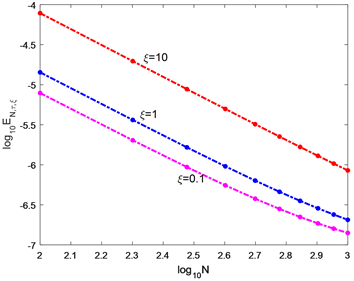Figure 1. ${\mathrm{log}}_{10}{E}_{N,\tau ,\xi }:\tau =0.001$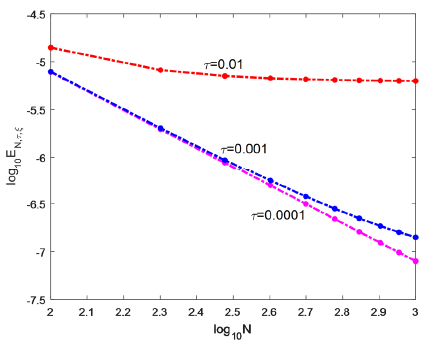Figure 2. ${\mathrm{log}}_{10}{E}_{N,\tau ,\xi }:\xi =0.1,\text{\hspace{0.17em}}T=1$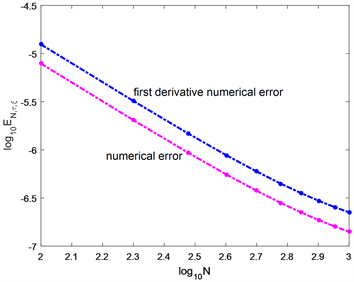Figure 3. ${\mathrm{log}}_{10}E{1}_{N,\tau ,\xi }:\xi =0.1,\text{\hspace{0.17em}}\tau =0.001$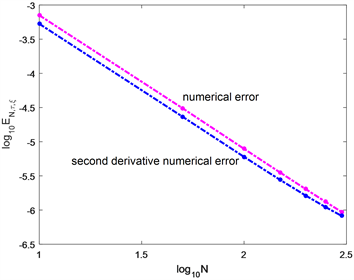Figure 4. ${\mathrm{log}}_{10}E{2}_{N,\tau ,\xi }:\xi =0.1,\text{\hspace{0.17em}}\tau =0.001$

$\left\{\begin{array}{l}{u}_{t}={u}_{xx}-2\mathrm{sin}\left(x\right){\text{e}}^{-3t},0 (12)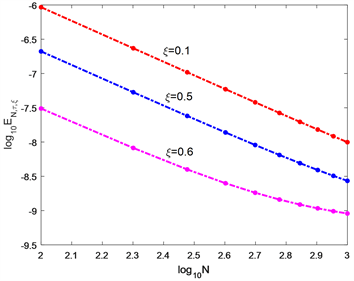Figure 5. ${\mathrm{log}}_{10}{E}_{N,\tau ,\xi }:\tau =0.0001$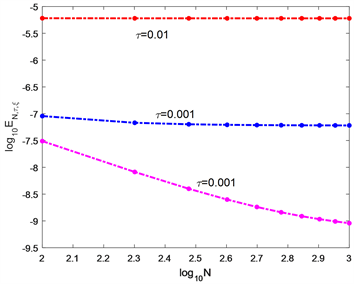Figure 6. ${\mathrm{log}}_{10}{E}_{N,\tau ,\xi }:\xi =0.6,\text{\hspace{0.17em}}T=1$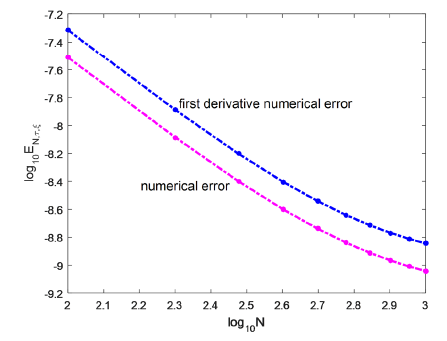Figure 7. ${\mathrm{log}}_{10}E{1}_{N,\tau ,\xi }:\xi =0.6,\text{\hspace{0.17em}}\tau =0.0001$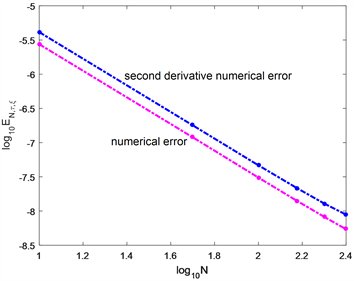Figure 8. ${\mathrm{log}}_{10}E{2}_{N,\tau ,\xi }:\xi =0.6,\text{\hspace{0.17em}}\tau =0.0001$

4. 结论

Cubic Spline Solution to Initial Boundary Value Problems of Heat Transfer Equations[J]. 应用数学进展, 2019, 08(08): 1375-1383. https://doi.org/10.12677/AAM.2019.88161

1. 1. 谷超豪, 李大潜, 陈恕行, 等. 数学物理方程[M]. 第三版. 北京: 高等教育出版社, 2002: 51-55.

2. 2. 李荣华, 刘播. 微分方程数值解法[M]. 第四版. 北京: 高等教育出版社, 2009: 107-145.

3. 3. 王天军, 贾丽蕊. 非线性热传导方程的Lagrange插值逼近[J]. 河南科技大学学报(自然科学版), 2011, 32(2): 68-71.

4. 4. 王天军, 李清, 殷艳红. 非线性热传导方程混合问题的插值逼近[J]. 航空计算技术, 2012, 42(2): 1-3.

5. 5. 杨继明. 热传导方程初边值问题的谱方法[J]. 湖南工程学院学报(自然科学版), 2007, 17(2): 71-73.

6. 6. 王天军. 半无界非线性热传导方程的Laguerre拟谱方法[J]. 应用数学与计算数学学报, 2013, 27(1): 9-15.

7. 7. Guo, B.Y. and Wang, T.J. (2006) Mixed Legendre-Hermite Spectral Method for Heat Transfer in an Infinite Plate. Computers & Mathematics with Applications, 51, 587-602. https://doi.org/10.1016/j.camwa.2006.03.004

8. 8. Arshad, K. (2004) Parametric Cubic Spline Solution of Two Point Boundary Value Problems. Applied Mathematics & Computation, 154, 175-182. https://doi.org/10.1016/S0096-3003(03)00701-X

9. 9. Wang, T.J., Zhou, Q.X. and Cui, T.T. (2015) Cubic Spline Solution for a Class of Boundary Value Problems Using Spectral Collocation Method. International Conference on Advanced Mechatronic Systems, Beijing, 22-24. https://doi.org/10.1109/ICAMechS.2015.7287095

10. 10. 柴果, 王天军. 非线性常微分方程边值问题的三次样条解[J]. 黑龙江大学自然科学报, 2017, 34(5): 544-548.